Aptitude Tests 4 Me

Non Verbal Logic

Detailed solution

96.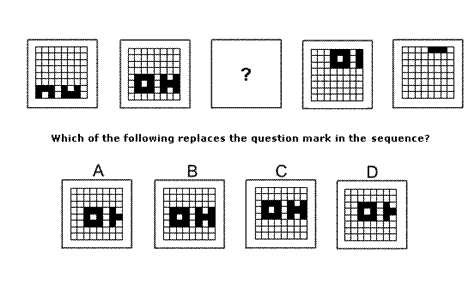Solution: D Explanation: In this question the squares making up the letters of the word OH move around the grid, moving one place to the right and two places up each time. Following this rule, the missing diagram of the sequence must be D.

97.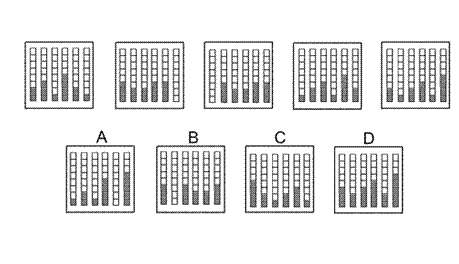Solution: B Explanation: In this question there are two rules to follow that are applied alternately. The first rule is that the numbers of grey squares in the odd-numbered columns increase by 1 while the numbers of grey squares in the even-numbered columns decrease by 1. The second rule is that the columns are all shifted one place to the right except the column on the right which moves to the position of the first column on the left. To find the next diagram of the sequence, therefore, the first rule should be applied next, and the correct answer is B.

98.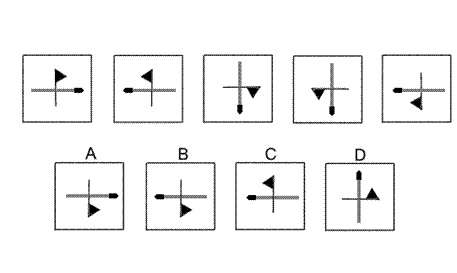Solution: A Explanation: In this question there is a flag and a matchstick that are reflected successively in two different axes. The first axis is the vertical line passing through the centre. The second axis is the diagonal line stretching from the bottom left corner to the top right corner. To obtain the next diagram of the sequence, therefore, the flag and matchstick should be reflected in the vertical axis, and the correct answer is A.

99.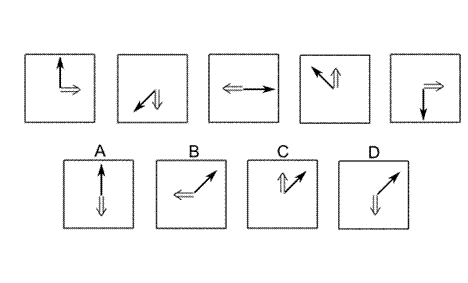Solution: D Explanation: In this question there are two different arrows - a single black arrow and a double grey arrow. Each arrow rotates according to its own rule. The black arrow rotates around the centre by 135° anticlockwise each time. Following this rule, the correct answer could be B, C or D. The double arrow rotates around the centre by 90° clockwise each time. Following this rule also, the next diagram of the sequence is D

100.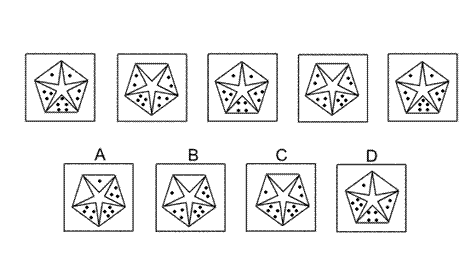Solution: C Explanation: In this question there are two rules to follow that are applied simultaneously. The first rule is that the pentagon and star rotates by 72° clockwise each time. The second rule concerns the numbers of dots in each of the five triangles. As the pentagon rotates: • If the triangle is moving downwards, then the number of dots in the triangle increases by 1. • If the triangle is moving upwards, then the number of dots in the triangle decreases by 1. When these two rules are applied simultaneously, the correct answer is C.

 1 2 3 4 5 6 7 8 9 10 11 12 13 14 15 16 17 18 19 20 21 22 23 24 25 26 27 28 29 30 31 32 33 34 35 36 37 38 39 40 41 42 43 44 45 46 47 48 49 50 51 52 53 54 55 56 57 58 59 60 61 62 63 64 65 66 67 68 69 70 71 72 73 74 75 76 77 78 79 80 81 82 83 84 85 86 87 88Passage ReadingVerbal LogicNon Verbal LogicNumerical LogicData InterpretationReasoningAnalytical AbilityBasic NumeracyAbout UsContactPrivacy PolicyMajor TestsFAQ